## Deviation Actions

This is a simulation which uses a cannon to calculate PI.

Given a circular pond in a square field where the pond and field have the same diameter, we fire a cannon into the field over and over, hitting randomly and count the number of times we hit the pond.

Because the area of a square is (W squared) where "W" is the width of the square and because the area of a circle is (PI times D squared) where "D" is the diameter of the circle, if a circle and square have the same width, the ratio of the area of the circle to the area of the square is PI / 4.

So we keep shooting cannonballs into the field and then we check the ratio of times we hit the pond divided by the times we fired in total. Take that ratio, multiply by 4 and we get our current guess at the value of PI. As the number of cannonballs fired increases, we should get closer and closer to PI.

Keep in mind that, while this is a valid method of calculating PI, it is also very inefficient as each additional digit of accuracy requires 10x as many shots as the previous digit.
Image details
Image size
500x550px 10.89 KB
Published:
© 2005 - 2021 DovSherman
Comments38
Join the community to add your comment. Already a deviant? Log Inhow long can this go on??!! I left it on left and came back and it was still shooting! Is there a limit??!!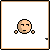Very nice. We'll keep working on the "random" bit. What did you use for this?As in, did you write the code for this? And what program did you use for the graphics?

I'm no computer guy, just mathy and physicsy. I am interested in what exactly you're running here...

I'm afraid my questions are vague because my understanding is similarly vague...Oh, I see. It's a very simple Flash-based program, written with Flash Actionscript. I made the graphics myself - the canon using Swift3D and the rest with the simple graphics editor built into Flash.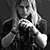isn't it somehow connected with the integral over the circular path?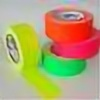Wow... Thats an interesting way to calculate pi. I wish we did something like this in my math class.Wow, How long does this thing go for? It's well over 2000 right now and still going!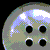things that make you go hmmm..
grrr math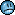Interesting... and a touch confusing.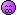No. My BA degree is in Animation. But I almost majored in Computer Science and I've been programming almost as long as I have been drawing. I took honors math classes in high school and was nominated to the NC School of Science and Math but just missed making it in on a technicality.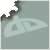Nice flash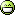Yay for probobility theories!

Request: speed up the time between cannon fires and see how long it takes to get the first 100 digits of piIf I open the dictionary to the word "geek", do I find your face?Close. A circle with a radius of R has an area of 2*Pi*(R squared). If the circle is the same width as a square, that square has a diameter of 2R and an area of (2R) squared.

So that's 2*Pi*R*R for a circle and 4*R*R for a square. If we factor out the R squared, we get a ratio of 2*Pi to 4.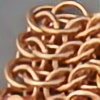What are you talking about? A circle with radius r has an area of Pi(r^2). But the radius is only half the width of the square, so the sides are 2r and the area is 4r^2.I thought the area of a circle was simply Pi r2, and the circumference formula was the one that required the coefficient of two (i.e. 2 Pi r). Then factoring out the r2 from your area equation you get a ratio of Pi: 4, which is what you have based your program on...
I'm sorry if I come across as... something. I'm just confused myself and trying to clarify =]Cool idea.
Never have heard of such a thing but now I know, kudos to you.I thought that if the cannon in thid deviation is alive cutely.
Join the community to add your comment. Already a deviant? Log In
©2021 DeviantArt
All Rights reserved